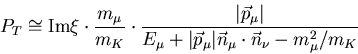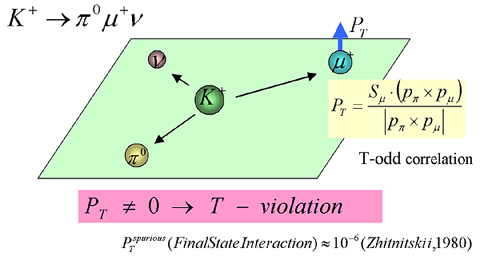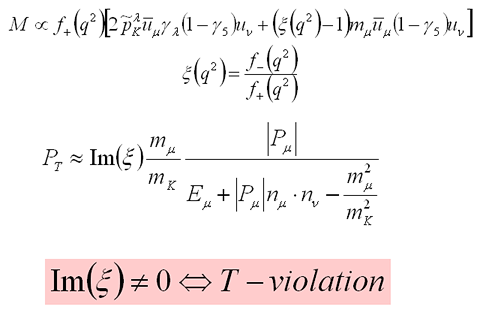## Transverse Muon Polarization and Time Reversal Invariance Violation

The transverse muon polarization (PT) in the K+ → π0μ+ν decay (K+μ3) is the pollarization compornet The transverse muon polarization normal to decay plane (PT) in the K+ → π0μ+ν decay is a T-odd correlation of PT = σμ• (pπ0 × pμ+) / |pπ0 × pμ+| . A non-vanishing PT would be a clear sign for T violation because the spurious effect from final state interactions is known to be negligibly small (order of magnitude of 10-6. The imaginary part of the ratio of two decay form factors ξ = f-(q2) / f+(q2) is responsible for T violation and PT can be written aswith Imξ and a kinematical factor. E246 is aiming for a sensitivity of about σ(Imξ) = 0.01. Under the assumption of CPT invariance, T violation provides a test of CP violation models. The most distinct feature here is the fact that the standard model has no experimentally detectable contribution up to a vertex correction contribution of ≈10-7. Thus, PT is a sensitive¡¡probe of new physics beyond the standard model. The very small final state interaction is in sharp contrast to KL→π-μ+ν (K0μ3) in which there is a spurious PT as large as 10-3. This gives a unique advantage to K+μ3 in the PT study. The history of PT experiments is long but there has been only one K+μ3 experiment performed before using an in-flight decay technique.